Ex 5.1

Chapter 5 Class 12 Continuity and Differentiability
Serial order wise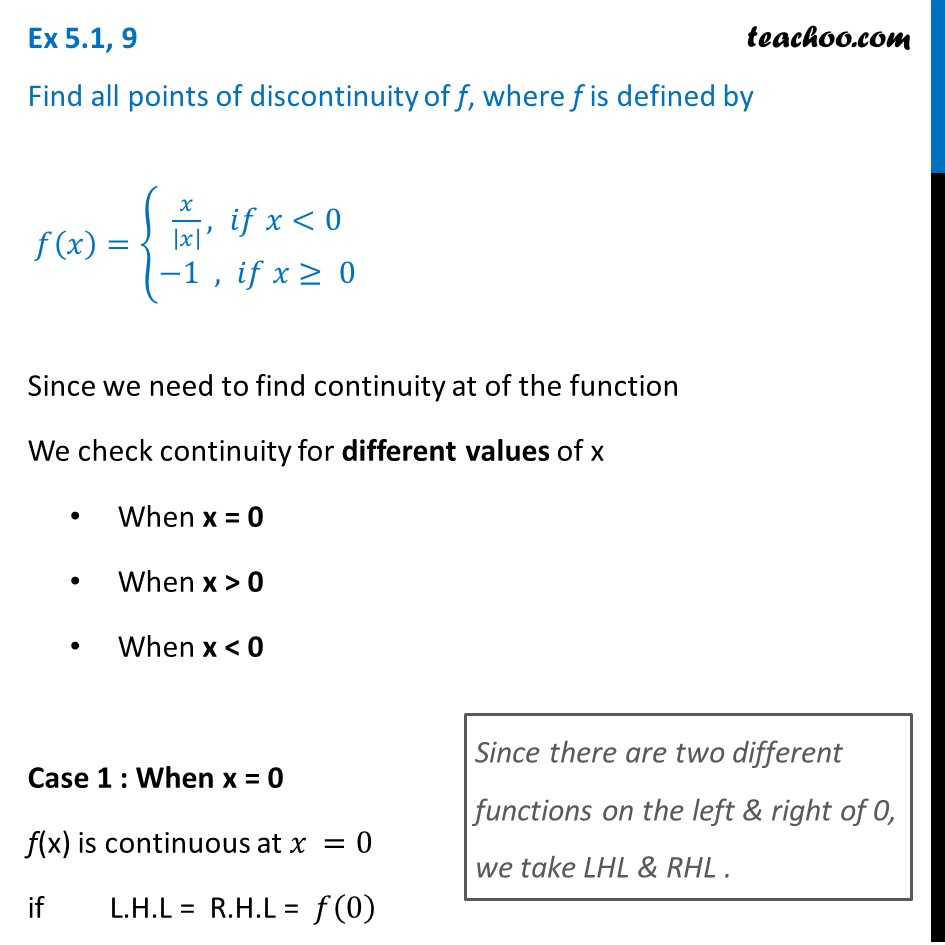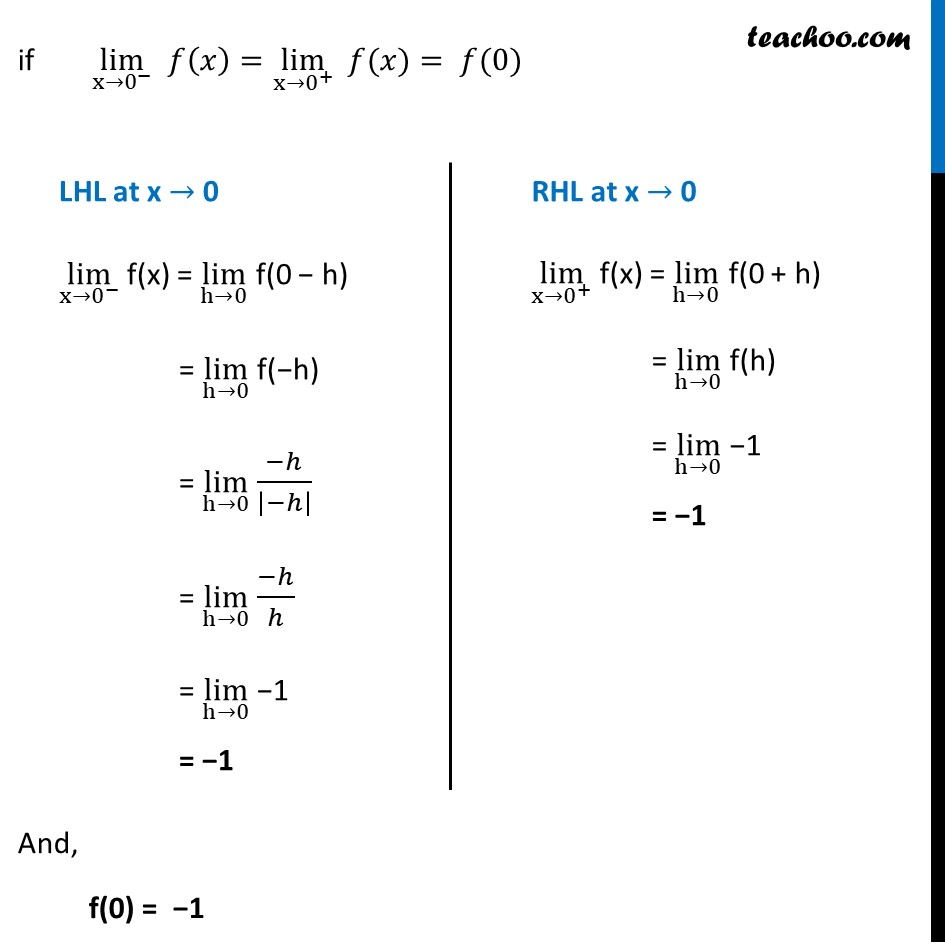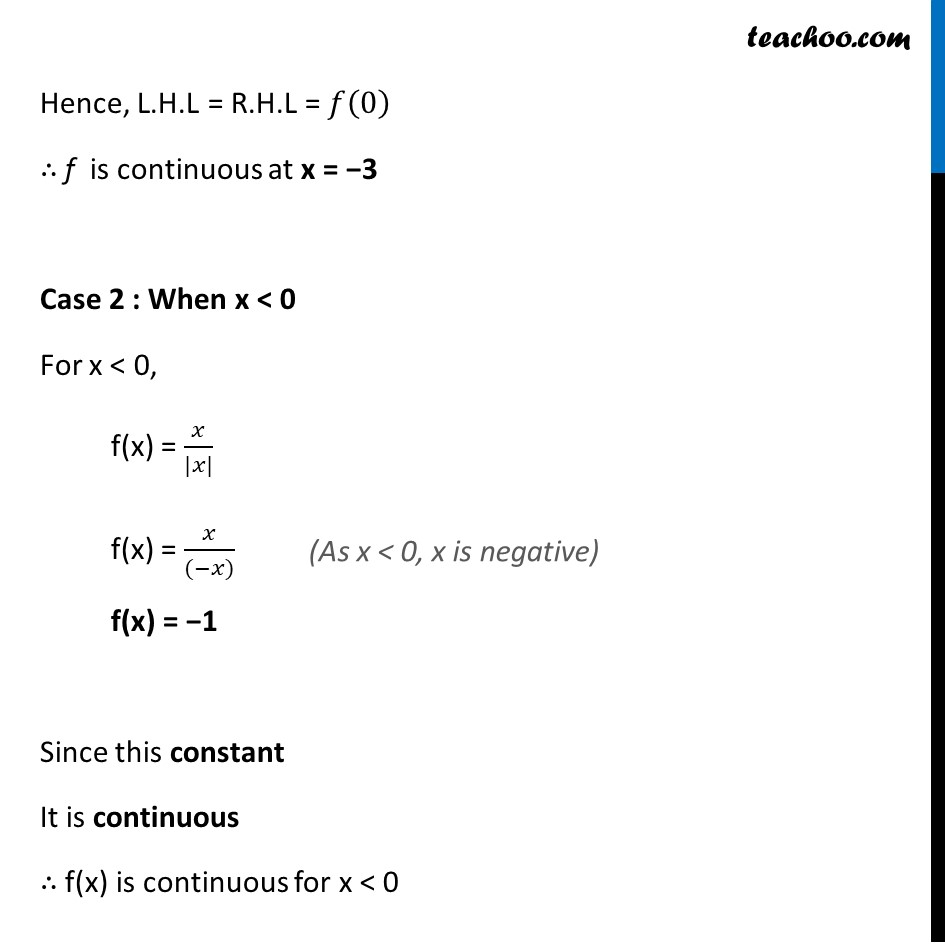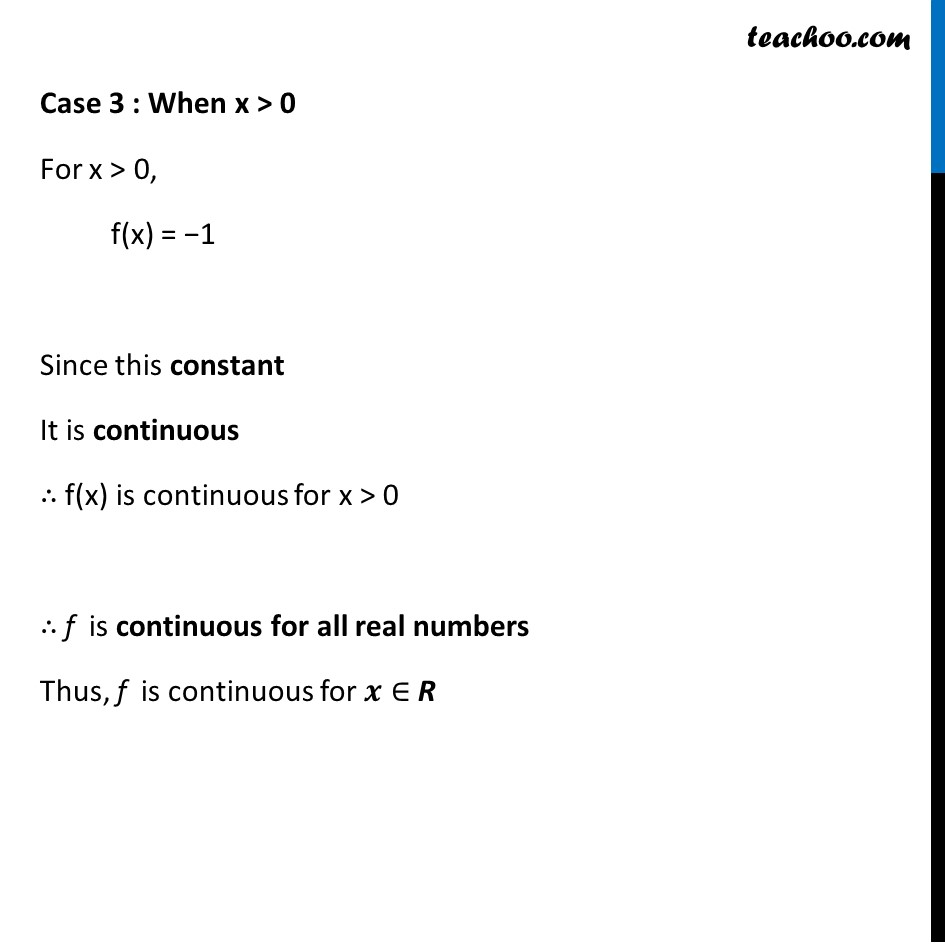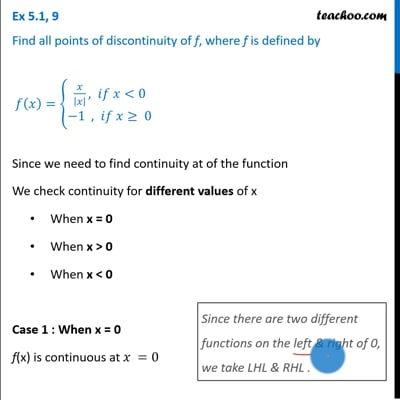This video is only available for Teachoo black users

Solve all your doubts with Teachoo Black (new monthly pack available now!)

### Transcript

Ex 5.1, 9 Find all points of discontinuity of f, where f is defined by 𝑓(𝑥)={█(𝑥/|𝑥| , 𝑖𝑓 𝑥<0@&−1 , 𝑖𝑓 𝑥≥ 0)┤ Since we need to find continuity at of the function We check continuity for different values of x When x = 0 When x > 0 When x < 0 Case 1 : When x = 0 f(x) is continuous at 𝑥 =0 if L.H.L = R.H.L = 𝑓(0) Since there are two different functions on the left & right of 0, we take LHL & RHL . if lim┬(x→0^− ) 𝑓(𝑥)=lim┬(x→0^+ ) " " 𝑓(𝑥)= 𝑓(0) And, f(0) = −1 LHL at x → 0 lim┬(x→0^− ) f(x) = lim┬(h→0) f(0 − h) = lim┬(h→0) f(−h) = lim┬(h→0) (−ℎ)/|−ℎ| = lim┬(h→0) (−ℎ)/ℎ = lim┬(h→0) −1 = −1 RHL at x → 0 lim┬(x→0^+ ) f(x) = lim┬(h→0) f(0 + h) = lim┬(h→0) f(h) = lim┬(h→0) −1 = −1 Hence, L.H.L = R.H.L = 𝑓(0) ∴ f is continuous at x = −3 Case 2 : When x < 0 For x < 0, f(x) = 𝑥/(|𝑥|) f(x) = 𝑥/((−𝑥)) f(x) = −1 Since this constant It is continuous ∴ f(x) is continuous for x < 0 (As x < 0, x is negative) Case 3 : When x > 0 For x > 0, f(x) = −1 Since this constant It is continuous ∴ f(x) is continuous for x > 0 ∴ f is continuous for all real numbers Thus, f is continuous for 𝒙∈ R